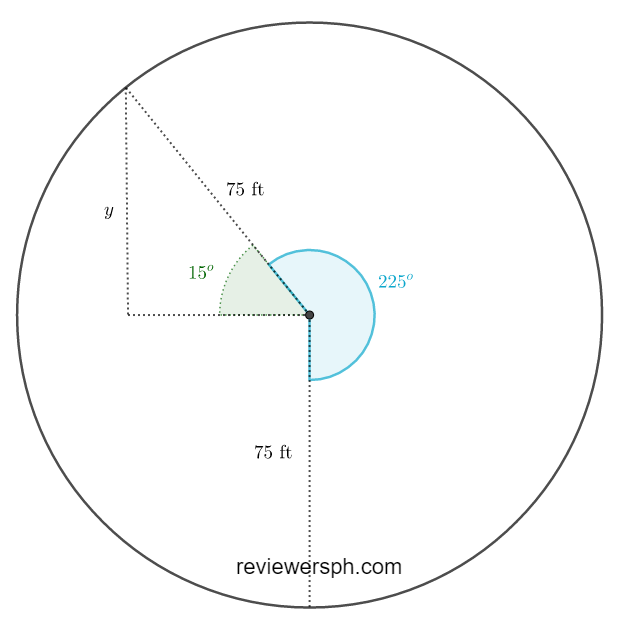### Math Notes

Subjects

#### Algebra Solutions

##### Topics || Problems

A Ferris wheel has a radius of 75 ft. You board a car at the bottom of the Ferris wheel, which is 10 feet above the ground, and rotate 255 degrees counterclockwise before the ride temporarily stops. How high above the ground are you when the ride stops? If the radius of the Ferris wheel is doubled, is your height above the ground doubled? Explain your reasoning.Let $$h$$ be the height of the car above the ground and $$y$$ be the height from the center of the Ferris wheel to the car after it sopped.

$$h = y +75+10$$

But, $$\sin 15 = \frac{y}{75}$$

$$y=75\sin 15$$

$$h =75\sin 15+75+10$$

$$h \approx 104.41~ft$$

After the Ferris wheel stops, the car is approximately $$104.41~\text{ft}$$ above the ground.

To double the height, means $$h_{\text{new}}=2h$$

$$2h = 2(y+10+75)$$

$$2h = 2y+2(10) +2(75))$$

In the equation $$h=y +10 + 75$$, if the radius is doubled, the new height would be $$h_{\text{new}} = 2y +10+2(75)$$.
Notice that $$10$$ is not doubled, and the new $$h$$ is not equal to the supposed doubled height.